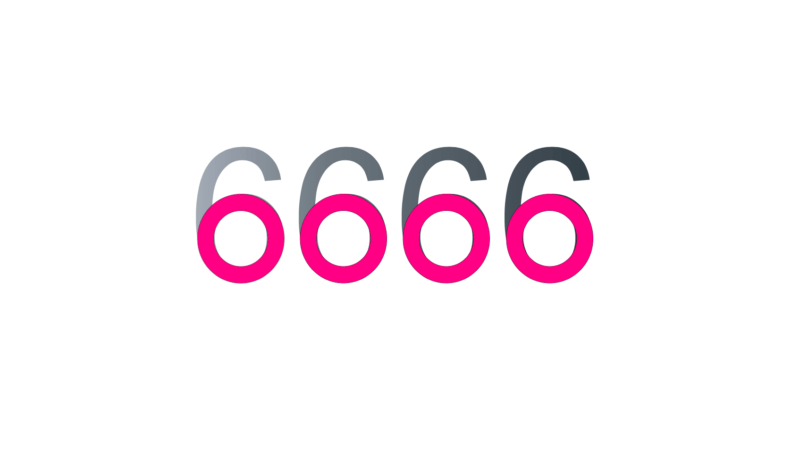SherlockHolmes576's Profile
Curious
36
points

Questions
1

4

SherlockHolmes576 loves solving puzzles at PuzzleFry.com. I am proud PuzzleFry member and like my time invested in solving brain teasers.
•Curious Asked on 30th April 2019 in

1700 KM

Distance (Code) = Sum of the letters as numbers
Note that:
Vowels = 300
Consonants = 200

Example:
America = A + M + E + R + I + C + A = 300 + 200 + 300 + 200 + 300 + 200 + 300 =1800 KM
Morocco = M + O + R + O + C + C + O = 200 + 300 + 200 + 300 + 200 + 200 + 300 = 1700 KM

• 2000 views
•Curious Asked on 30th April 2019 in1 pink circle = every circle you see in the number

• 14636 views
•Curious Asked on 30th April 2019 in

Today
Tomorrow

• 16641 views
•Curious Asked on 29th April 2019 in

82709

If we label each digit a, b, c, d and e, we get the following equations.
1. b + c = 9
2. a = b3
3. c + e = 16 (since 16 is the smallest number with five divisors – 1, 2, 4, 8 and 16).
4. d = 6 * d (The fourth and second-to-last digits are the same number, meaning d must be zero to satisfy the equation)
5. b must be zero, one or two (b can’t be three because that makes a=27, which isn’t a single digit). Zero and one result in duplicate digits (00907 and 11808 respectively) so the only remaining value for b is 2, giving us 82709 and warmth.

• 2966 views